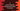# C program to count and print frequency of each letter in a word# C program to count and print frequency of each letter in a word :

In this tutorial, we will learn how to count and print the frequency of each letter in a word in C programming. Our program will take the input from the user, calculate and print out the frequency of each character in the word one by one. We will use for-loop to calculate the frequency. Before going to show you the program, let me explain how the algorithm works :

## Algorithm :

1. Ask the user to enter a string, read it and store it in a char array.
2. Create one int array of size 256 to hold the frequency of the characters. Assign 0 to each element of the array.

So, we have one integer array of size 256 with value 0 for each array position. Our program will read the string and update this array. The program will increment the ASCII position of a character in the array. For example, if our string is AAA, it will increment the position 65 of the array three times, because ASCII value of A is 65. 3. As explained above, scan the array one by one character and increment its corresponding position in the array by 1. 4. After the input array is scanned, read the ASCII value and count holding array one by one. If any value is more than 0, print out the result to the user.

I hope that you have got the main logic behind this program. The main advantage of using an extra array is that it will require only one time to scan the input array.

## C program :

``````#include <stdio.h>
#include <stdlib.h>

//1
#define MAX_STRING_SIZE 30
#define ASCII_ARRAY_SIZE 256

//2
int checkIfValidChar(char ch)
{
if ((ch >= 'a' && ch <= 'z') || (ch >= 'A' && ch <= 'Z'))
{
return 1;
}
return 0;
}

int main()
{
//3
char userInput[MAX_STRING_SIZE];
int charArray[ASCII_ARRAY_SIZE] = {0};
int i;

//4
printf("Enter a string : ");

//5
fgets(userInput, MAX_STRING_SIZE, stdin);

//6
for (i = 0; i < sizeof(userInput) / sizeof(userInput); i++)
{
charArray[userInput[i]]++;
}

//7
for (i = 0; i < sizeof(charArray) / sizeof(charArray); i++)
{

//8
char ch = i;
if (charArray[i] != 0 && checkIfValidChar(ch) == 1)
{
printf("%c appeared for %d time\n", i, charArray[i]);
}
}
return 0;
}
``````

### Explanation :

_The commented numbers in the above program denote the step numbers below : _

1. MAX_STRING_SIZE is defined as 30. This is the maximum string size user can enter. ASCII_ARRAY_SIZE is the size of the array holding the count of each character, i.e. it should be the size of the total ASCII characters, i.e. 256
2. checkIfValidChar method is used to check if a character is valid or not, i.e. if it is only an alphabet, we are considering it as valid. You can also add one more && operation to include the condition for a numerical value.
3. First of all, create two arrays: one char array to hold the user input line and one int array to hold the count of each character of the string. We have initialized each element of this array as 0.
4. Ask the user to enter a string.
5. Read it and store it in the char array we have created at the start of the program.
6. Run one for loop to scan each character of the array one by one. Increment its ASCII indexed value of charArray by 1 on each time. e.g. if the current character is of ASCII value 68 increment charArray by 1.
7. After the first for loop is completed, run one for loop to scan the current values of the array elements. If any value is not zero, that means the character of ASCII for that index was in the string.
8. Check if the character is valid or not if the value of any position in charArray is not zero. If valid, print out the result.

### Sample Output :

``````Enter a string : Hello
H appeared for 1 time
e appeared for 1 time
l appeared for 2 time
o appeared for 1 time

Enter a string : Hello World
H appeared for 1 time
W appeared for 1 time
d appeared for 1 time
e appeared for 1 time
l appeared for 3 time
o appeared for 2 time
r appeared for 1 time

Enter a string : abcd
a appeared for 1 time
b appeared for 1 time
c appeared for 1 time
d appeared for 1 time
``````

### Conclusion :

In this tutorial, we have learned how to find out the number of occurance for each character in a string in C. Try to implement the above program on your machine and drop one comment below if you have any queries.

You might also like :